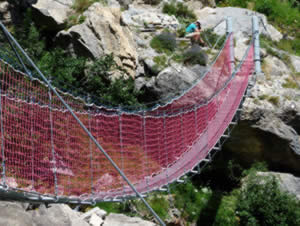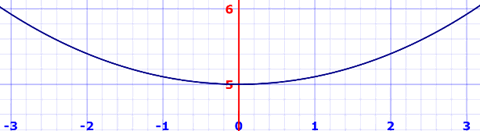# Hyperbolic Functions

The two basic hyperbolic functions are "sinh" and "cosh":

### Hyperbolic Sine:

sinh(x) = ex − e−x 2

(pronounced "shine")

### Hyperbolic Cosine:

cosh(x) = ex + e−x 2

(pronounced "cosh")

They use the natural exponential function ex

And are not the same as sin(x) and cos(x), but a little bit similar:sinh vs sincosh vs cos## Catenary

One of the interesting uses of Hyperbolic Functions is the curve made by suspended cables or chains.

A hanging cable forms a curve called a catenary defined using the cosh function:

f(x) = a cosh(x/a)

Like in this example from the page arc length :## Other Hyperbolic Functions

From sinh and cosh we can create:

### Hyperbolic tangent "tanh" (pronounced "than"):

tanh(x) = sinh(x) cosh(x) = ex − e−x ex + e−xtanh vs tan

### Hyperbolic cotangent:

coth(x) = cosh(x) sinh(x) = ex + e−x ex − e−x

### Hyperbolic secant:

sech(x) = 1 cosh(x) = 2 ex + e−x

### Hyperbolic cosecant "csch" or "cosech":

csch(x) = 1 sinh(x) = 2 ex − e−x

## Why the Word "Hyperbolic" ?

Because it comes from measurements made on a Hyperbola:So, just like the trigonometric functions relate to a circle, the hyperbolic functions relate to a hyperbola.

## Identities

• sinh(−x) = −sinh(x)
• cosh(−x) = cosh(x)

And

• tanh(−x) = −tanh(x)
• coth(−x) = −coth(x)
• sech(−x) = sech(x)
• csch(−x) = −csch(x)

## Odd and Even

Both cosh and sech are Even Functions, the rest are Odd Functions.

## Derivatives

Derivatives are:

d dx sinh(x) = cosh(x)

d dx cosh(x) = sinh(x)

d dx tanh(x) = 1 − tanh2(x)